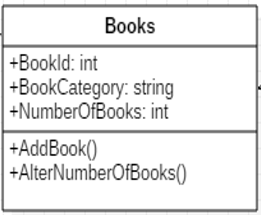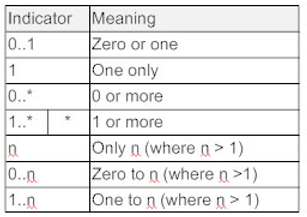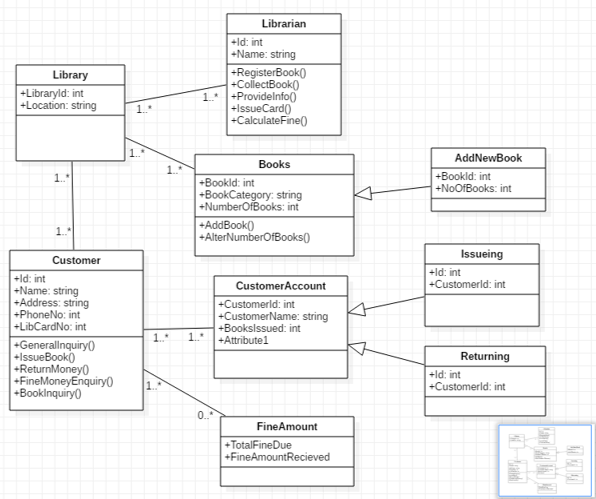# The class diagram | Software Engineering

The Class diagram of the software. In this article, we are going to briefly discuss about this diagram, regarding why is it used and what are the features and specifications of this diagram, and also about how it is represented. We will also discuss about the various relations that each class of the model hold with other classes.
Submitted by Monika Sharma, on October 19, 2019

Classes are used to represent the object-oriented models. As we know that in the OOPs structure, every entity is treated as an object. A class thus can be defined as a collection of objects that share the common properties and behavior.

The Class diagram of the software consists of all the classes that exist in the software. In the class diagram, a class is represented via a rectangular box. Each of the classes is divided into three sections. The topmost section consists of the class name. The second section consists of the names of all the attributes that the class contains with their data types. And the third section consists of the methods (or functions) that are defined in the class. (It must be noted that the section of attributes and methods can be empty, but the class name cannot.)

For example: Consider a class named "books" which has some attributes and methods. The class diagram for this very class would look something like this:Here, Books is the name of the class, which has the following attributes: BookId of integer type, BookCategory of String type, NumberOfBooks of integer type, and has two methods namely: AddBook() and AlterNumberOfBooks().

Now, the class diagram not only consists of the classes, but these classes also hold some relation with each other, and this relation is also represented in this diagram.

A class is connected with another class (with whom it holds a relation) using a single line, and on that line, we represent the cardinality. The cardinality represents the number of entities that exist in the relation. It may be one to one, one to many, etc. The cardinality symbols are represented as follows for a class:Image source: https://wcs.smartdraw.com/class-diagram/img/notes.jpg?bn=1510011152

Example:

Let us consider the example of the library management system. The following is the class diagram for the same.

It consists of nine classes that are interconnected. The relations and cardinality of each of them can also be inferred from the class diagram itself.Class Diagram for library management System